﻿ Molecular dynamics calculation for equilibrium state of water - XSim

# Molecular dynamics calculation for equilibrium state of water

Update: December 28, 2017
OpenFOAM 4.x

## Case directory

\$FOAM_TUTORIALS/discreteMethods/molecularDynamics/mdEquilibrationFoam/periodicCubeWater

## Summary

Molecular Dynamics (MD) calculation is performed to calculate the equilibrium state of water.

The properties of the particles are defined in the files "moleculeProperties" and "idList" in the directory "constant". The external force and interparticle potential are defined in the file "potentialDict" in the directory "system", the initial conditions are defined in the file "mdInitialiseDict", and the temperature at equilibrium is defined in the file "mdEquilibrationDict".

The size of the model in the X, Y, and Z directions is 4.22 nm, respectively, and each face is set to a periodic boundary condition.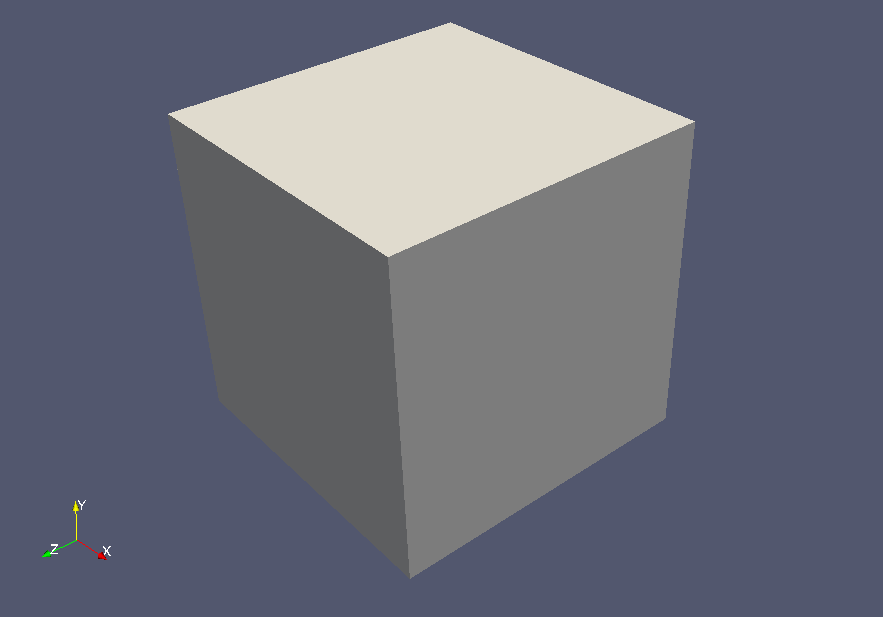Model geometry

The initial position of the particles is defined based on the mesh. The mesh is as follows.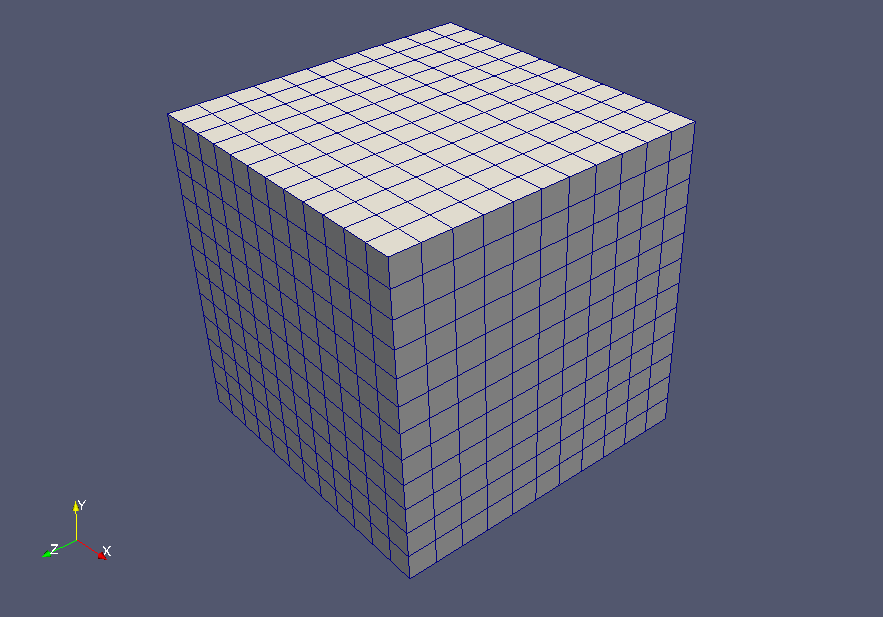Meshes

The calculation result is as follows.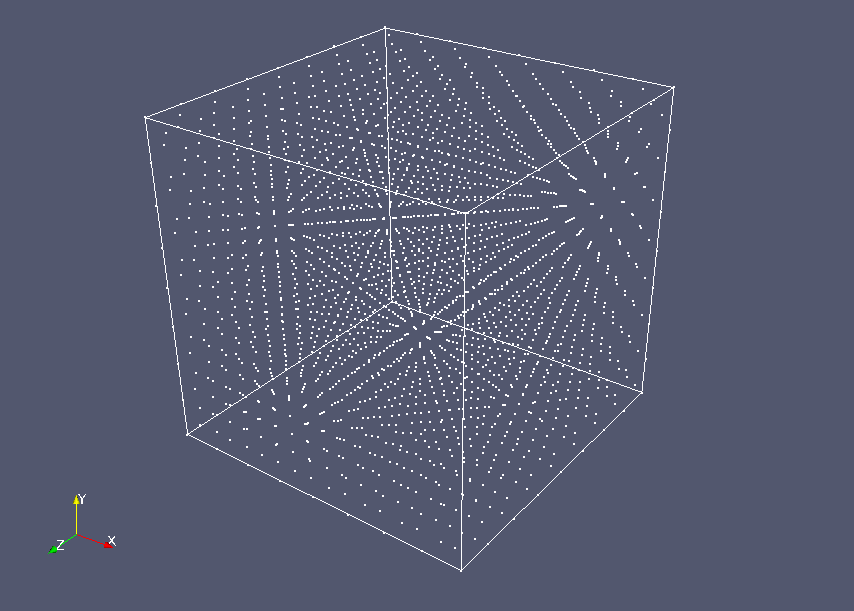Particle distribution at 0 sec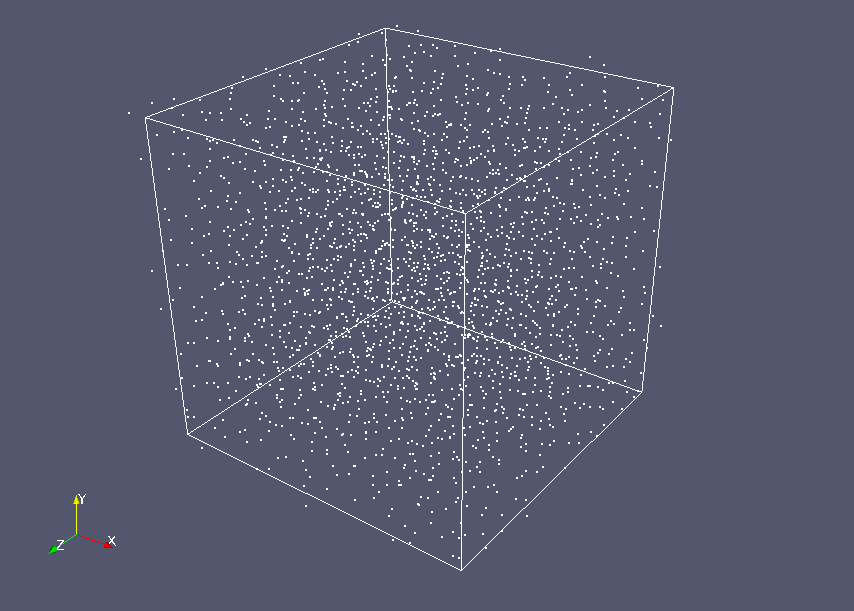Particle distribution at 5.0×10-12 sec

The energy and force of each potential at each distance are output to the file "O-O" and the file "electrostatic". In the file, the first column is the distance, the second column is the energy, and the third column is the force.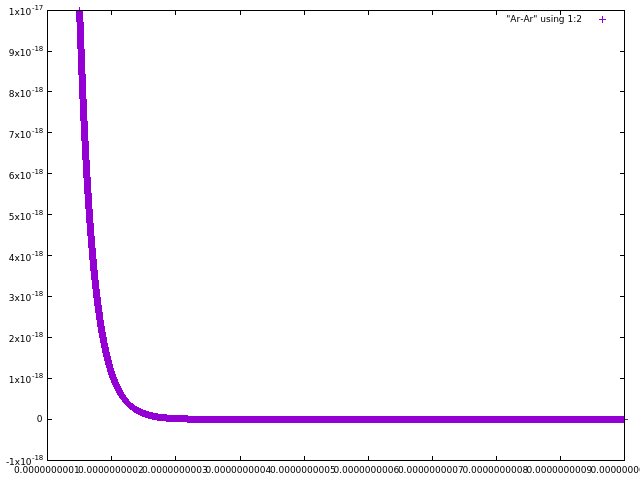Results of distance-energy calculations are output to file "O-O"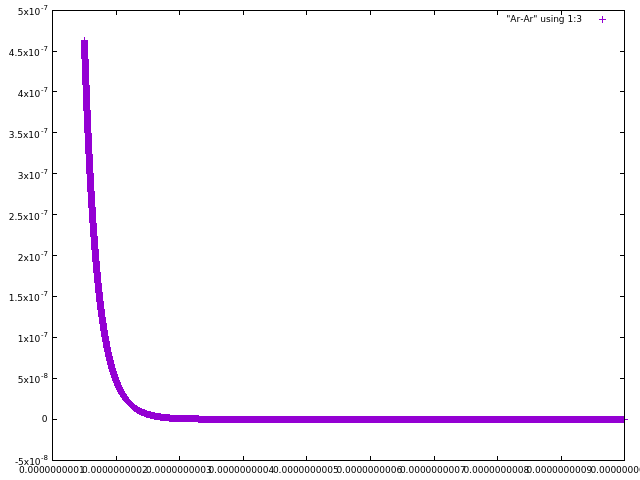Results of distance-force calculations are output to file "O-O"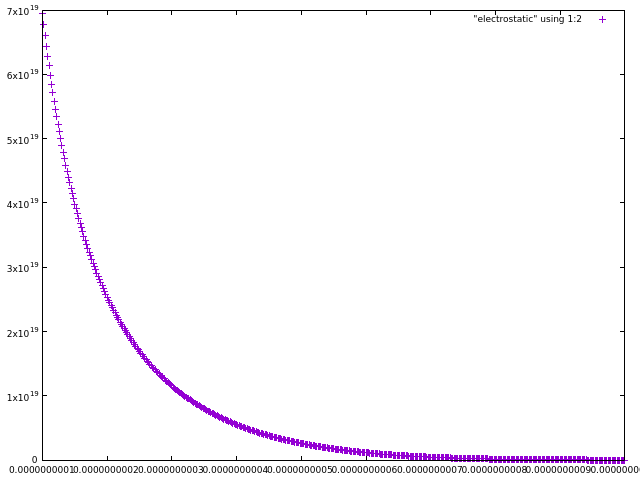Results of distance-energy calculations are output to file "electrostatic"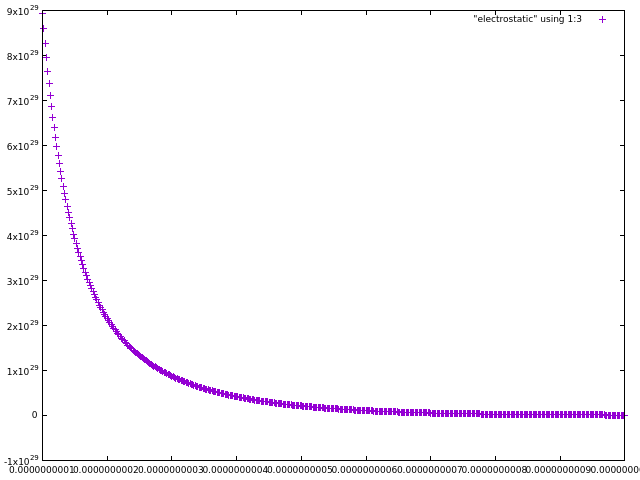Results of distance-force calculations are output to file "electrostatic"

## Visualization operations

To visualize discrete particles, load the data into ParaView by the paraFoam command, and then set the following in the Properties tab of the pipeline browser and click the Apply button.

• Check only "moleculeCloud" in the Mesh Parts list
• Uncheck all items in Volume Fields list
• Check all items in Lagrangian Fields list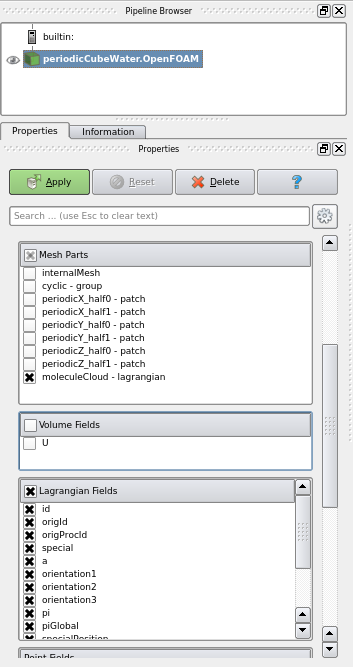Select display items

## Commands

cp -r \$FOAM_TUTORIALS/discreteMethods/molecularDynamics/mdEquilibrationFoam/periodicCubeWater periodicCubeWater
cd periodicCubeWater

blockMesh
mdInitialise
mdEquilibrationFoam

paraFoam

gnuplot
gnuplot> plot "O-O" using 1:2
gnuplot> plot "O-O" using 1:3
gnuplot> plot "electrostatic" using 1:2
gnuplot> plot "electrostatic" using 1:3

## Calculation time

43 minutes 37.74 seconds *Single, Inter(R) Core(TM) i7-2600 CPU @ 3.40GHz 3.40GHz